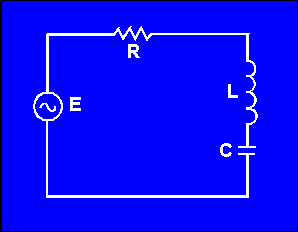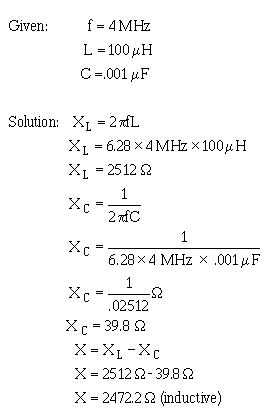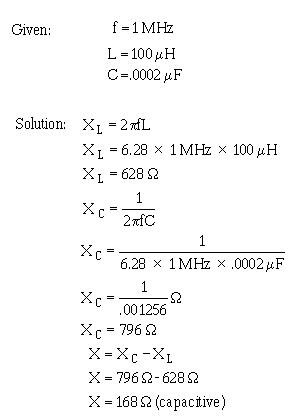capacitance have been explained individually in ac circuits. The rest of this chapter will concern the combination of inductance, capacitance, and resistance in ac circuits. To explain the various properties that exist within ac circuits, the series RLC circuit will be used. Figure 4-4 is the schematic diagram of the series RLC circuit. The symbol shown in figure 4-4 that is marked E is the general symbol used to indicate an ac voltage source. ">Custom SearchREACTANCE, IMPEDANCE, AND POWER RELATIONSHIPS IN AC CIRCUITS Up to this point inductance and capacitance have been explained individually in ac circuits. The rest of this chapter will concern the combination of inductance, capacitance, and resistance in ac circuits. To explain the various properties that exist within ac circuits, the series RLC circuit will be used. Figure 4-4 is the schematic diagram of the series RLC circuit. The symbol shown in figure 4-4 that is marked E is the general symbol used to indicate an ac voltage source. Figure 4 - 4. - Series RLC circuit.REACTANCE The effect of inductive reactance is to cause the current to lag the voltage, while that of capacitive reactance is to cause the current to lead the voltage. Therefore, since inductive reactance and capacitive reactance are exactly opposite in their effects, what will be the result when the two are combined? It is not hard to see that the net effect is a tendency to cancel each other, with the combined effect then equal to the difference between their values. This resultant is called REACTANCE; it is represented by the symbol X; and expressed by the equation X = XL - XC or X = XC - X L. Thus, if a circuit contains 50 ohms of inductive reactance and 25 ohms of capacitive reactance in series, the net reactance, or X, is 50 ohms - 25 ohms, or 25 ohms of inductive reactance. For a practical example, suppose you have a circuit containing an inductor of 100 mH in series with a capacitor of .001 mF, and operating at a frequency of 4 MHz. What is the value of net reactance, or X?Now assume you have a circuit containing a 100 - mH inductor in series with a .0002-mF capacitor, and operating at a frequency of 1 MHz. What is the value of the resultant reactance in this case?You will notice that in this case the inductive reactance is smaller than the capacitive reactance and is therefore subtracted from the capacitive reactance. These two examples serve to illustrate an important point: when capacitive and inductive reactance are combined in series, the smaller is always subtracted from the larger and the resultant reactance always takes the characteristics of the larger. Q.13 What is the formula for determining total reactance in a series circuit where the values of XC and X L are known? Q.14 What is the total amount of reactance (X) in a series circuit which contains an XL of 20 ohms and an X C of 50 ohms? (Indicate whether X is capacitive or inductive)Integrated Publishing, Inc. - A (SDVOSB) Service Disabled Veteran Owned Small Business